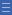## Featured resource

Home > Topdrawer > Mental computation > Activities > Back through ten

# Back through ten

Back through ten

Back-through-ten strategies involve using decades as landmarks to solve harder calculations. For example, 52 – 8 = ? can be solved by calculating 52 – 2 = 50 then 50 – 6 = 44.

The back-through-ten strategy tends to be more difficult than the up-through-ten strategy because it involves breaking down numbers, as opposed to building them up. However, the same key knowledge of facts to 10 and place value is required. You can watch the Back through Ten video.

You can download the Back through Ten video transcript.

Back-through-ten strategies can be practised using the learning object L97 The takeaway bar: make your own easy subtractions

These ideas are further explored in Place Value and Number Facts: Addition and Subtraction.

Yes

Yes

Name Class SectionYear 3: Recall addition facts for single-digit numbers and related subtraction facts to develop increasingly efficient mental strategies for computa... Infobox 3Year 2: Explore the connection between addition and subtraction Infobox 3Year 2: Solve simple addition and subtraction problems using a range of efficient mental and written strategies Infobox 3Source Infobox 3